QRectF

QSizeF

QRect¶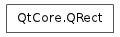Detailed Description¶

The PySide.QtCore.QRect class defines a rectangle in the plane using integer precision.

A rectangle is normally expressed as an upper-left corner and a size. The size (width and height) of a PySide.QtCore.QRect is always equivalent to the mathematical rectangle that forms the basis for its rendering.

A PySide.QtCore.QRect can be constructed with a set of left, top, width and height integers, or from a PySide.QtCore.QPoint and a PySide.QtCore.QSize . The following code creates two identical rectangles.

r1 = QRect(100, 200, 11, 16)
r2 = QRect(QPoint(100, 200), QSize(11, 16))

There is a third constructor that creates a PySide.QtCore.QRect using the top-left and bottom-right coordinates, but we recommend that you avoid using it. The rationale is that for historical reasons the values returned by the PySide.QtCore.QRect.bottom() and PySide.QtCore.QRect.right() functions deviate from the true bottom-right corner of the rectangle.

The PySide.QtCore.QRect class provides a collection of functions that return the various rectangle coordinates, and enable manipulation of these. PySide.QtCore.QRect also provide functions to move the rectangle relative to the various coordinates. In addition there is a PySide.QtCore.QRect.moveTo() function that moves the rectangle, leaving its top left corner at the given coordinates. Alternatively, the PySide.QtCore.QRect.translate() function moves the rectangle the given offset relative to the current position, and the PySide.QtCore.QRect.translated() function returns a translated copy of this rectangle.

The PySide.QtCore.QRect.size() function returns the rectange’s dimensions as a PySide.QtCore.QSize . The dimensions can also be retrieved separately using the PySide.QtCore.QRect.width() and PySide.QtCore.QRect.height() functions. To manipulate the dimensions use the PySide.QtCore.QRect.setSize() , PySide.QtCore.QRect.setWidth() or PySide.QtCore.QRect.setHeight() functions. Alternatively, the size can be changed by applying either of the functions setting the rectangle coordinates, for example, PySide.QtCore.QRect.setBottom() or PySide.QtCore.QRect.setRight() .

The PySide.QtCore.QRect.contains() function tells whether a given point is inside the rectangle or not, and the PySide.QtCore.QRect.intersects() function returns true if this rectangle intersects with a given rectangle. The PySide.QtCore.QRect class also provides the PySide.QtCore.QRect.intersected() function which returns the intersection rectangle, and the PySide.QtCore.QRect.united() function which returns the rectangle that encloses the given rectangle and this:PySide.QtCore.QRect.intersected() PySide.QtCore.QRect.united()

The PySide.QtCore.QRect.isEmpty() function returns true if PySide.QtCore.QRect.left() > PySide.QtCore.QRect.right() or PySide.QtCore.QRect.top() > PySide.QtCore.QRect.bottom() . Note that an empty rectangle is not valid: The PySide.QtCore.QRect.isValid() function returns true if PySide.QtCore.QRect.left() <= PySide.QtCore.QRect.right() andPySide.QtCore.QRect.top() <= PySide.QtCore.QRect.bottom() . A null rectangle ( PySide.QtCore.QRect.isNull() == true) on the other hand, has both width and height set to 0.

Note that due to the way PySide.QtCore.QRect and PySide.QtCore.QRectF are defined, an empty PySide.QtCore.QRect is defined in essentially the same way as PySide.QtCore.QRectF .

Finally, PySide.QtCore.QRect objects can be streamed as well as compared.

Rendering¶

When using an anti-aliased painter, the boundary line of a PySide.QtCore.QRect will be rendered symmetrically on both sides of the mathematical rectangle’s boundary line. But when using an aliased painter (the default) other rules apply.

Then, when rendering with a one pixel wide pen the PySide.QtCore.QRect ‘s boundary line will be rendered to the right and below the mathematical rectangle’s boundary line.

When rendering with a two pixels wide pen the boundary line will be split in the middle by the mathematical rectangle. This will be the case whenever the pen is set to an even number of pixels, while rendering with a pen with an odd number of pixels, the spare pixel will be rendered to the right and below the mathematical rectangle as in the one pixel case.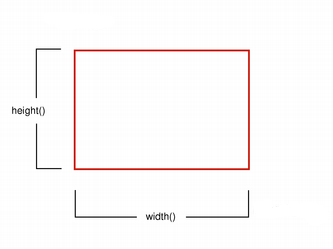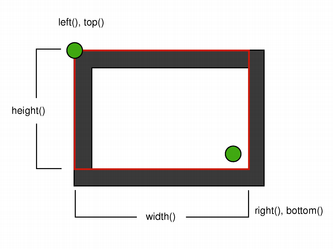Logical representation One pixel wide pen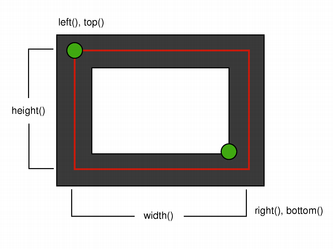Two pixel wide pen Three pixel wide pen

Coordinates¶

The PySide.QtCore.QRect class provides a collection of functions that return the various rectangle coordinates, and enable manipulation of these. PySide.QtCore.QRect also provide functions to move the rectangle relative to the various coordinates.

For example the PySide.QtCore.QRect.left() , PySide.QtCore.QRect.setLeft() and PySide.QtCore.QRect.moveLeft() functions as an example: PySide.QtCore.QRect.left() returns the x-coordinate of the rectangle’s left edge, PySide.QtCore.QRect.setLeft() sets the left edge of the rectangle to the given x coordinate (it may change the width, but will never change the rectangle’s right edge) and PySide.QtCore.QRect.moveLeft() moves the entire rectangle horizontally, leaving the rectangle’s left edge at the given x coordinate and its size unchanged.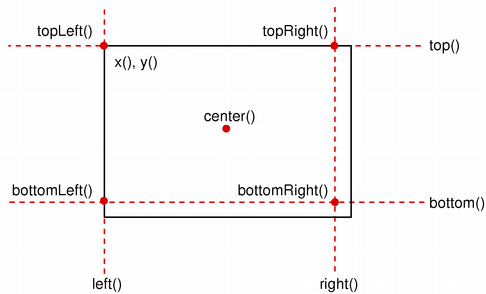Note that for historical reasons the values returned by the PySide.QtCore.QRect.bottom() and PySide.QtCore.QRect.right() functions deviate from the true bottom-right corner of the rectangle: The PySide.QtCore.QRect.right() function returns * PySide.QtCore.QRect.left() + :meth:`PySide.QtCore.QRect.width` *- 1 and the PySide.QtCore.QRect.bottom() function returns * PySide.QtCore.QRect.top() + :meth:`PySide.QtCore.QRect.height` *- 1 . The same is the case for the point returned by the PySide.QtCore.QRect.bottomRight() convenience function. In addition, the x and y coordinate of the PySide.QtCore.QRect.topRight() and PySide.QtCore.QRect.bottomLeft() functions, respectively, contain the same deviation from the true right and bottom edges.

We recommend that you use PySide.QtCore.QRect.x() + PySide.QtCore.QRect.width() and PySide.QtCore.QRect.y() + PySide.QtCore.QRect.height() to find the true bottom-right corner, and avoid PySide.QtCore.QRect.right() and PySide.QtCore.QRect.bottom() . Another solution is to use PySide.QtCore.QRectF : The PySide.QtCore.QRectF class defines a rectangle in the plane using floating point accuracy for coordinates, and the QRectF.right() and QRectF.bottom() functions do return the right and bottom coordinates.

It is also possible to add offsets to this rectangle’s coordinates using the PySide.QtCore.QRect.adjust() function, as well as retrieve a new rectangle based on adjustments of the original one using the PySide.QtCore.QRect.adjusted() function. If either of the width and height is negative, use the PySide.QtCore.QRect.normalized() function to retrieve a rectangle where the corners are swapped.

In addition, PySide.QtCore.QRect provides the PySide.QtCore.QRect.getCoords() function which extracts the position of the rectangle’s top-left and bottom-right corner, and the PySide.QtCore.QRect.getRect() function which extracts the rectangle’s top-left corner, width and height. Use the PySide.QtCore.QRect.setCoords() and PySide.QtCore.QRect.setRect() function to manipulate the rectangle’s coordinates and dimensions in one go.

class PySide.QtCore.QRect
class PySide.QtCore.QRect(topleft, bottomright)
class PySide.QtCore.QRect(topleft, size)
class PySide.QtCore.QRect(QRect)
class PySide.QtCore.QRect(left, top, width, height)
Parameters: width – PySide.QtCore.int size – PySide.QtCore.QSize topleft – PySide.QtCore.QPoint left – PySide.QtCore.int height – PySide.QtCore.int bottomright – PySide.QtCore.QPoint QRect – PySide.QtCore.QRect top – PySide.QtCore.int

Constructs a null rectangle.

Constructs a rectangle with the given topLeft and bottomRight corners.

Constructs a rectangle with the given topLeft corner and the given size .

Constructs a rectangle with (x , y ) as its top-left corner and the given width and height .

PySide.QtCore.QRect.__reduce__()
Return type: PyObject
PySide.QtCore.QRect.__repr__()
Return type: PyObject
Parameters: x1 – PySide.QtCore.int y1 – PySide.QtCore.int x2 – PySide.QtCore.int y2 – PySide.QtCore.int

Adds dx1 , dy1 , dx2 and dy2 respectively to the existing coordinates of the rectangle.

Parameters: x1 – PySide.QtCore.int y1 – PySide.QtCore.int x2 – PySide.QtCore.int y2 – PySide.QtCore.int PySide.QtCore.QRect

Returns a new rectangle with dx1 , dy1 , dx2 and dy2 added respectively to the existing coordinates of this rectangle.

PySide.QtCore.QRect.bottom()
Return type: PySide.QtCore.int

Returns the y-coordinate of the rectangle’s bottom edge.

Note that for historical reasons this function returns PySide.QtCore.QRect.top() + PySide.QtCore.QRect.height() - 1; use PySide.QtCore.QRect.y() + PySide.QtCore.QRect.height() to retrieve the true y-coordinate.

PySide.QtCore.QRect.bottomLeft()
Return type: PySide.QtCore.QPoint

Returns the position of the rectangle’s bottom-left corner. Note that for historical reasons this function returns PySide.QtCore.QPoint ( PySide.QtCore.QRect.left() , PySide.QtCore.QRect.top() + PySide.QtCore.QRect.height() - 1).

PySide.QtCore.QRect.bottomRight()
Return type: PySide.QtCore.QPoint

Returns the position of the rectangle’s bottom-right corner.

Note that for historical reasons this function returns PySide.QtCore.QPoint ( PySide.QtCore.QRect.left() + PySide.QtCore.QRect.width() -1, PySide.QtCore.QRect.top() + PySide.QtCore.QRect.height() - 1).

PySide.QtCore.QRect.center()
Return type: PySide.QtCore.QPoint

Returns the center point of the rectangle.

PySide.QtCore.QRect.contains(x, y, proper)
Parameters: x – PySide.QtCore.int y – PySide.QtCore.int proper – PySide.QtCore.bool PySide.QtCore.bool

Returns true if the point (x , y ) is inside or on the edge of the rectangle, otherwise returns false. If proper is true, this function only returns true if the point is entirely inside the rectangle(not on the edge).

PySide.QtCore.QRect.contains(x, y)
Parameters: x – PySide.QtCore.int y – PySide.QtCore.int PySide.QtCore.bool

Returns true if the point (x , y ) is inside this rectangle, otherwise returns false.

PySide.QtCore.QRect.contains(r[, proper=false])
Parameters: r – PySide.QtCore.QRect proper – PySide.QtCore.bool PySide.QtCore.bool

Returns true if the given rectangle is inside this rectangle. otherwise returns false. If proper is true, this function only returns true if the rectangle is entirely inside this rectangle (not on the edge).

PySide.QtCore.QRect.contains(p[, proper=false])
Parameters: p – PySide.QtCore.QPoint proper – PySide.QtCore.bool PySide.QtCore.bool

Returns true if the given point is inside or on the edge of the rectangle, otherwise returns false. If proper is true, this function only returns true if the given point is inside the rectangle (i.e., not on the edge).

PySide.QtCore.QRect.getCoords()

Extracts the position of the rectangle’s top-left corner to *``x1`` and *``y1`` , and the position of the bottom-right corner to *``x2`` and *``y2`` .

PySide.QtCore.QRect.getRect()

Extracts the position of the rectangle’s top-left corner to *``x`` and *``y`` , and its dimensions to *``width`` and *``height`` .

PySide.QtCore.QRect.height()
Return type: PySide.QtCore.int

Returns the height of the rectangle.

PySide.QtCore.QRect.intersect(r)
Parameters: r – PySide.QtCore.QRect PySide.QtCore.QRect

PySide.QtCore.QRect.intersected(other)
Parameters: other – PySide.QtCore.QRect PySide.QtCore.QRect

Returns the intersection of this rectangle and the given rectangle . Note that r.intersected(s) is equivalent to r & s .PySide.QtCore.QRect.intersects() PySide.QtCore.QRect.united() PySide.QtCore.QRect.operator&=()

PySide.QtCore.QRect.intersects(r)
Parameters: r – PySide.QtCore.QRect PySide.QtCore.bool

Returns true if this rectangle intersects with the given rectangle (i.e., there is at least one pixel that is within both rectangles), otherwise returns false.

The intersection rectangle can be retrieved using the PySide.QtCore.QRect.intersected() function.

PySide.QtCore.QRect.isEmpty()
Return type: PySide.QtCore.bool

Returns true if the rectangle is empty, otherwise returns false.

An empty rectangle has a PySide.QtCore.QRect.left() > PySide.QtCore.QRect.right() or PySide.QtCore.QRect.top() > PySide.QtCore.QRect.bottom() . An empty rectangle is not valid (i.e., PySide.QtCore.QRect.isEmpty() == ! PySide.QtCore.QRect.isValid() ).

Use the PySide.QtCore.QRect.normalized() function to retrieve a rectangle where the corners are swapped.

PySide.QtCore.QRect.isNull()
Return type: PySide.QtCore.bool

Returns true if the rectangle is a null rectangle, otherwise returns false.

A null rectangle has both the width and the height set to 0 (i.e., PySide.QtCore.QRect.right() == PySide.QtCore.QRect.left() - 1 and PySide.QtCore.QRect.bottom() == PySide.QtCore.QRect.top() - 1). A null rectangle is also empty, and hence is not valid.

PySide.QtCore.QRect.isValid()
Return type: PySide.QtCore.bool

Returns true if the rectangle is valid, otherwise returns false.

A valid rectangle has a PySide.QtCore.QRect.left() < PySide.QtCore.QRect.right() and PySide.QtCore.QRect.top() < PySide.QtCore.QRect.bottom() . Note that non-trivial operations like intersections are not defined for invalid rectangles. A valid rectangle is not empty (i.e., PySide.QtCore.QRect.isValid() == ! PySide.QtCore.QRect.isEmpty() ).

PySide.QtCore.QRect.left()
Return type: PySide.QtCore.int

Returns the x-coordinate of the rectangle’s left edge. Equivalent to PySide.QtCore.QRect.x() .

PySide.QtCore.QRect.moveBottom(pos)
Parameters: pos – PySide.QtCore.int

Moves the rectangle vertically, leaving the rectangle’s bottom edge at the given y coordinate. The rectangle’s size is unchanged.

PySide.QtCore.QRect.moveBottomLeft(p)
Parameters: p – PySide.QtCore.QPoint

Moves the rectangle, leaving the bottom-left corner at the given position . The rectangle’s size is unchanged.

PySide.QtCore.QRect.moveBottomRight(p)
Parameters: p – PySide.QtCore.QPoint

Moves the rectangle, leaving the bottom-right corner at the given position . The rectangle’s size is unchanged.

PySide.QtCore.QRect.moveCenter(p)
Parameters: p – PySide.QtCore.QPoint

Moves the rectangle, leaving the center point at the given position . The rectangle’s size is unchanged.

PySide.QtCore.QRect.moveLeft(pos)
Parameters: pos – PySide.QtCore.int

Moves the rectangle horizontally, leaving the rectangle’s left edge at the given x coordinate. The rectangle’s size is unchanged.

PySide.QtCore.QRect.moveRight(pos)
Parameters: pos – PySide.QtCore.int

Moves the rectangle horizontally, leaving the rectangle’s right edge at the given x coordinate. The rectangle’s size is unchanged.

PySide.QtCore.QRect.moveTo(x, t)
Parameters: x – PySide.QtCore.int t – PySide.QtCore.int

Moves the rectangle, leaving the top-left corner at the given position (x , y ). The rectangle’s size is unchanged.

PySide.QtCore.QRect.moveTo(p)
Parameters: p – PySide.QtCore.QPoint

Moves the rectangle, leaving the top-left corner at the given position .

PySide.QtCore.QRect.moveTop(pos)
Parameters: pos – PySide.QtCore.int

Moves the rectangle vertically, leaving the rectangle’s top edge at the given y coordinate. The rectangle’s size is unchanged.

PySide.QtCore.QRect.moveTopLeft(p)
Parameters: p – PySide.QtCore.QPoint

Moves the rectangle, leaving the top-left corner at the given position . The rectangle’s size is unchanged.

PySide.QtCore.QRect.moveTopRight(p)
Parameters: p – PySide.QtCore.QPoint

Moves the rectangle, leaving the top-right corner at the given position . The rectangle’s size is unchanged.

PySide.QtCore.QRect.normalized()
Return type: PySide.QtCore.QRect

Returns a normalized rectangle; i.e., a rectangle that has a non-negative width and height.

If PySide.QtCore.QRect.width() < 0 the function swaps the left and right corners, and it swaps the top and bottom corners if PySide.QtCore.QRect.height() < 0.

PySide.QtCore.QRect.__ne__(arg__2)
Parameters: arg__2 – PySide.QtCore.QRect PySide.QtCore.bool
PySide.QtCore.QRect.__and__(r)
Parameters: r – PySide.QtCore.QRect PySide.QtCore.QRect

Returns the intersection of this rectangle and the given rectangle . Returns an empty rectangle if there is no intersection.

PySide.QtCore.QRect.operator&=() PySide.QtCore.QRect.intersected()

PySide.QtCore.QRect.__iand__(r)
Parameters: r – PySide.QtCore.QRect PySide.QtCore.QRect

Intersects this rectangle with the given rectangle .

PySide.QtCore.QRect.intersected() PySide.QtCore.QRect.operator&()

PySide.QtCore.QRect.__eq__(arg__2)
Parameters: arg__2 – PySide.QtCore.QRect PySide.QtCore.bool
PySide.QtCore.QRect.__or__(r)
Parameters: r – PySide.QtCore.QRect PySide.QtCore.QRect

Returns the bounding rectangle of this rectangle and the given rectangle .

PySide.QtCore.QRect.operator|=() PySide.QtCore.QRect.united()

PySide.QtCore.QRect.__ior__(r)
Parameters: r – PySide.QtCore.QRect PySide.QtCore.QRect

Unites this rectangle with the given rectangle .

PySide.QtCore.QRect.united() PySide.QtCore.QRect.operator|()

PySide.QtCore.QRect.right()
Return type: PySide.QtCore.int

Returns the x-coordinate of the rectangle’s right edge.

Note that for historical reasons this function returns PySide.QtCore.QRect.left() + PySide.QtCore.QRect.width() - 1; use PySide.QtCore.QRect.x() + PySide.QtCore.QRect.width() to retrieve the true x-coordinate.

PySide.QtCore.QRect.setBottom(pos)
Parameters: pos – PySide.QtCore.int

Sets the bottom edge of the rectangle to the given y coordinate. May change the height, but will never change the top edge of the rectangle.

PySide.QtCore.QRect.setBottomLeft(p)
Parameters: p – PySide.QtCore.QPoint

Set the bottom-left corner of the rectangle to the given position . May change the size, but will never change the top-right corner of the rectangle.

PySide.QtCore.QRect.setBottomRight(p)
Parameters: p – PySide.QtCore.QPoint

Set the bottom-right corner of the rectangle to the given position . May change the size, but will never change the top-left corner of the rectangle.

PySide.QtCore.QRect.setCoords(x1, y1, x2, y2)
Parameters: x1 – PySide.QtCore.int y1 – PySide.QtCore.int x2 – PySide.QtCore.int y2 – PySide.QtCore.int

Sets the coordinates of the rectangle’s top-left corner to (x1 , y1 ), and the coordinates of its bottom-right corner to (x2 , y2 ).

PySide.QtCore.QRect.setHeight(h)
Parameters: h – PySide.QtCore.int

Sets the height of the rectangle to the given height . The bottom edge is changed, but not the top one.

PySide.QtCore.QRect.setLeft(pos)
Parameters: pos – PySide.QtCore.int

Sets the left edge of the rectangle to the given x coordinate. May change the width, but will never change the right edge of the rectangle.

Equivalent to PySide.QtCore.QRect.setX() .

PySide.QtCore.QRect.setRect(x, y, w, h)
Parameters: x – PySide.QtCore.int y – PySide.QtCore.int w – PySide.QtCore.int h – PySide.QtCore.int

Sets the coordinates of the rectangle’s top-left corner to (x , y ), and its size to the given width and height .

PySide.QtCore.QRect.setRight(pos)
Parameters: pos – PySide.QtCore.int

Sets the right edge of the rectangle to the given x coordinate. May change the width, but will never change the left edge of the rectangle.

PySide.QtCore.QRect.setSize(s)
Parameters: s – PySide.QtCore.QSize

Sets the size of the rectangle to the given size . The top-left corner is not moved.

PySide.QtCore.QRect.setTop(pos)
Parameters: pos – PySide.QtCore.int

Sets the top edge of the rectangle to the given y coordinate. May change the height, but will never change the bottom edge of the rectangle.

Equivalent to PySide.QtCore.QRect.setY() .

PySide.QtCore.QRect.setTopLeft(p)
Parameters: p – PySide.QtCore.QPoint

Set the top-left corner of the rectangle to the given position . May change the size, but will never change the bottom-right corner of the rectangle.

PySide.QtCore.QRect.setTopRight(p)
Parameters: p – PySide.QtCore.QPoint

Set the top-right corner of the rectangle to the given position . May change the size, but will never change the bottom-left corner of the rectangle.

PySide.QtCore.QRect.setWidth(w)
Parameters: w – PySide.QtCore.int

Sets the width of the rectangle to the given width . The right edge is changed, but not the left one.

PySide.QtCore.QRect.setX(x)
Parameters: x – PySide.QtCore.int

Sets the left edge of the rectangle to the given x coordinate. May change the width, but will never change the right edge of the rectangle.

Equivalent to PySide.QtCore.QRect.setLeft() .

PySide.QtCore.QRect.setY(y)
Parameters: y – PySide.QtCore.int

Sets the top edge of the rectangle to the given y coordinate. May change the height, but will never change the bottom edge of the rectangle.

Equivalent to PySide.QtCore.QRect.setTop() .

PySide.QtCore.QRect.size()
Return type: PySide.QtCore.QSize

Returns the size of the rectangle.

PySide.QtCore.QRect.top()
Return type: PySide.QtCore.int

Returns the y-coordinate of the rectangle’s top edge. Equivalent to PySide.QtCore.QRect.y() .

PySide.QtCore.QRect.topLeft()
Return type: PySide.QtCore.QPoint

Returns the position of the rectangle’s top-left corner.

PySide.QtCore.QRect.topRight()
Return type: PySide.QtCore.QPoint

Returns the position of the rectangle’s top-right corner.

Note that for historical reasons this function returns PySide.QtCore.QPoint ( PySide.QtCore.QRect.left() + PySide.QtCore.QRect.width() -1, PySide.QtCore.QRect.top() ).

PySide.QtCore.QRect.translate(dx, dy)
Parameters: dx – PySide.QtCore.int dy – PySide.QtCore.int

Moves the rectangle dx along the x axis and dy along the y axis, relative to the current position. Positive values move the rectangle to the right and down.

PySide.QtCore.QRect.translate(p)
Parameters: p – PySide.QtCore.QPoint

Moves the rectangle offset . PySide.QtCore.QPoint.x() along the x axis and offset . PySide.QtCore.QPoint.y() along the y axis, relative to the current position.

PySide.QtCore.QRect.translated(p)
Parameters: p – PySide.QtCore.QPoint PySide.QtCore.QRect

Returns a copy of the rectangle that is translated offset . PySide.QtCore.QPoint.x() along the x axis and offset . PySide.QtCore.QPoint.y() along the y axis, relative to the current position.

PySide.QtCore.QRect.translated(dx, dy)
Parameters: dx – PySide.QtCore.int dy – PySide.QtCore.int PySide.QtCore.QRect

Returns a copy of the rectangle that is translated dx along the x axis and dy along the y axis, relative to the current position. Positive values move the rectangle to the right and down.

PySide.QtCore.QRect.unite(r)
Parameters: r – PySide.QtCore.QRect PySide.QtCore.QRect

PySide.QtCore.QRect.united(other)
Parameters: other – PySide.QtCore.QRect PySide.QtCore.QRect

Returns the bounding rectangle of this rectangle and the given rectangle .PySide.QtCore.QRect.width()
Return type: PySide.QtCore.int

Returns the width of the rectangle.

PySide.QtCore.QRect.x()
Return type: PySide.QtCore.int

Returns the x-coordinate of the rectangle’s left edge. Equivalent to PySide.QtCore.QRect.left() .

PySide.QtCore.QRect.y()
Return type: PySide.QtCore.int

Returns the y-coordinate of the rectangle’s top edge. Equivalent to PySide.QtCore.QRect.top() .